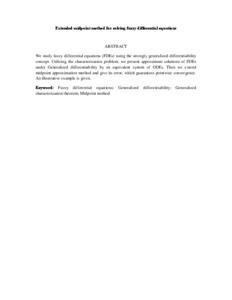# Extended midpoint method for solving fuzzy differential equations

## Citation

Afsharinafar, Reza and Ismail, Fudziah and Hosseini, Seyedali Ahmadian (2012) Extended midpoint method for solving fuzzy differential equations. In: 2012 IEEE Symposium on Humanities, Science and Engineering Research (SHUSER 2012), 24-27 June 2012, Renaissance Hotel, Kuala Lumpur. (pp. 1579-1584).

## Abstract

We study fuzzy differential equations (FDEs) using the strongly generalized differentiability concept. Utilizing the characterization problem, we present approximate solutions of FDEs under Generalized differentiability by an equivalent system of ODEs. Then we extend midpoint approximation method and give its error, which guarantees pointwise convergence. An illustrative example is given.Preview
Text (Abstract)
Extended midpoint method for solving fuzzy differential equations.pdfView Item